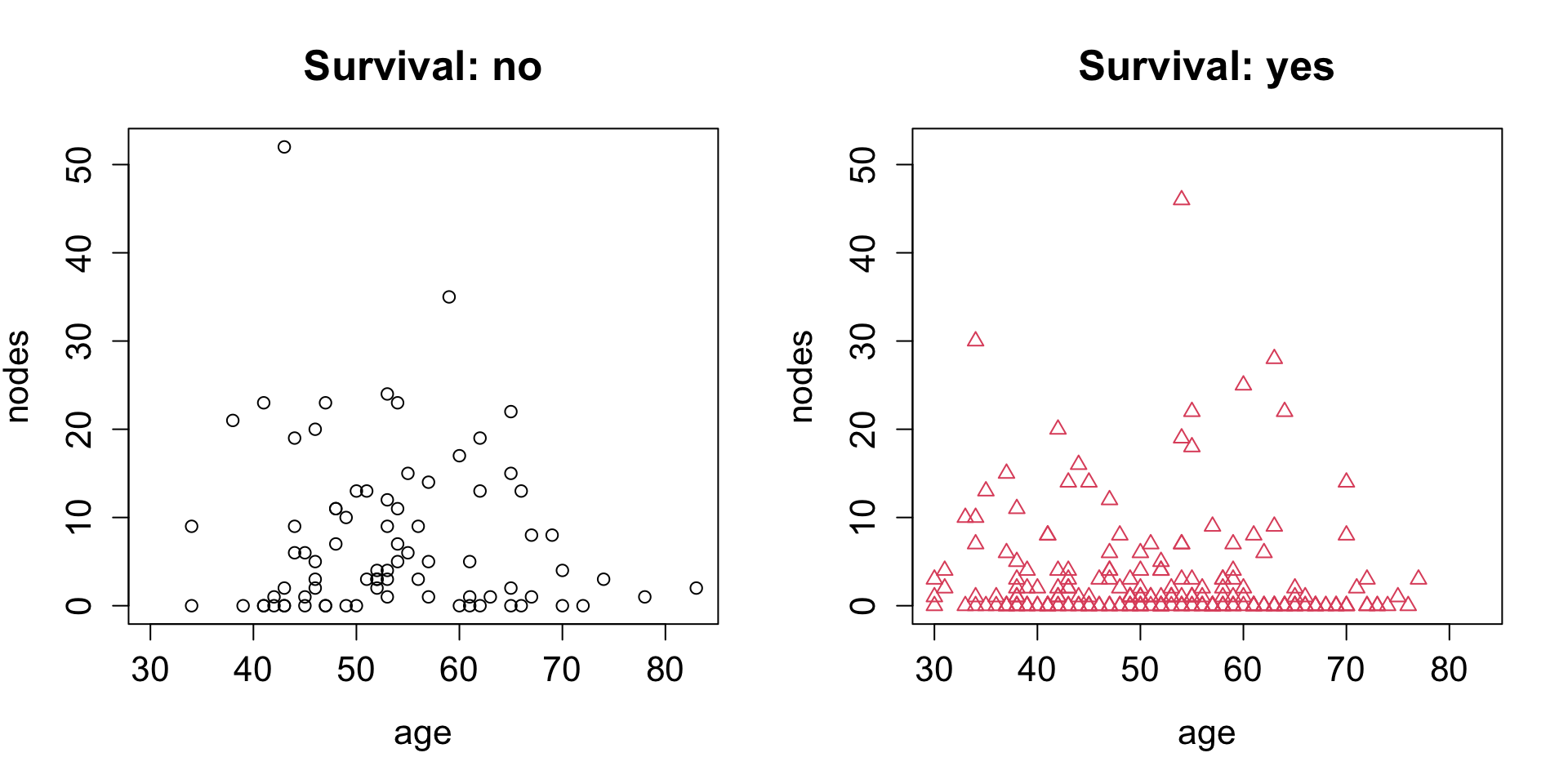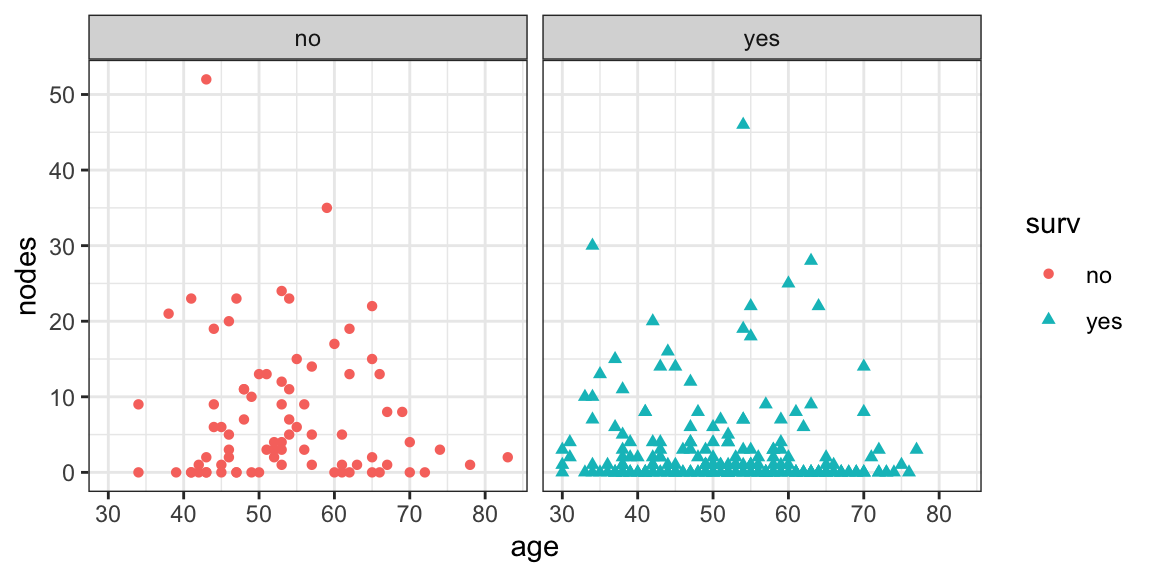# Preface

One of the first steps in the analysis of a new dataset, often as part of data cleaning, typically involves generation of high level summaries, such as: the numbers of observations and attributes (variables), which variables are predictors and which ones are (could be?) outcomes; what the ranges, distributions, and percentages of missing values in all the varianbles are; the strength of correlation among the predictors and between the predictors and the outcome(s), etc. It is usually at this stage when we develop our initial intuition about the level of difficulty of the problem and the challenges presented by this particular dataset. This is when (and how) we form our first set of ideas as to how to approach the problem. There are many multivariate methods under unsupervised learning umbrella that are extremely useful in this setting (which will be introduced later in the course), but first things first, and here we will start by loading few datasets into R and exploring their attributes in the form of univariate (e.g. single-variable) summaries and bivariate (two-variable) plots and contingency tables (where applicable).

For this problem set we will use datasets available from the UCI machine learning repository or subsets thereof cleaned up and pre-processed for the instructional purposes. For convenience and in order to avoid the dependence on UCI ML repository availability, we have copied the datasets into the course Canvas website. Once you have downloaded the data onto your computer, they can be imported into R using function `read.table` with necessary options (of which most useful/relevant include: `sep` – defining field separator and `header` – letting `read.table` know whether the first line in the text file contains the column names or the first row of data). In principle, `read.table` can also use URL as a full path to the dataset, but here, to be able to work independently of network connection and because of the pre-processing involved, you will need to download those datasets from Canvas website to your local computer and use `read.table` with appropriate paths to the local files. The simplest thing is probably to copy the data to the same directory where your .Rmd file is, in which case just the file name passed to `read.table` should suffice. As always, please remember, that `help(read.table)` (or, `?read.table` as a shorthand) will tell you quite a bit about this function and its parameters.

For those datasets that do not have column names included in their data files, it is often convenient to assign them explicitly. Please note that for some of these datasets categorical variables are encoded in the form of integer values (e.g. 1, 2, 3 and 4) and thus R will interpret those as continuous variables by default, while the behavior of many R functions depends on the type of the input variables (continuous vs categorical/factor).

The code excerpts and their output presented below illustrate some of these most basic steps as applied to one of the datasets available from UCI. The homework problems follow after that – they will require you to apply similar kind of approaches to generate high levels summaries of few other datasets that you are provided here with.

## Haberman Survival Dataset

Note how the `summary` function computes a 5-number summary for continuous variable, cannot do anything particularly useful for a general vector of strings, and counts the numbers of occurrences of distinct levels for a categorical variable (explicitly defined as a factor).

``````habDat <- read.table("~/Desktop/haberman.data",sep=",")
colnames(habDat) <- c("age","year","nodes","surv")
summary(habDat\$surv)``````
``````##    Min. 1st Qu.  Median    Mean 3rd Qu.    Max.
##   1.000   1.000   1.000   1.265   2.000   2.000``````
``````habDat\$surv <- c("yes","no")[habDat\$surv]
summary(habDat\$surv)``````
``````##    Length     Class      Mode
##       306 character character``````
``````habDat\$surv <- factor(habDat\$surv)
summary(habDat\$surv)``````
``````##  no yes
##  81 225``````

Below we demonstrate xy-scatterplots of two variables (patient’s age and node count), with color indicating their survival past 5 years. The first example uses basic plotting capabilities in R, while the second one shows how the same result can be achieved with `ggplot2` package. Note that in this particular example we choose to show the data stratified by the survival categorical variable in two separate scatterplots, side by side. The reason is purely aesthetic one: since we do indicate distinct classes of patients with different colors it would be entirely possible (and meaningful) to put all the data into a single scatterplot, same way it was done in class. However, the data at hand do not readily separate into (visually) distinct groups, at least in the projection on the two variables chosen here. There would be too much overplotting (exacerbated by the fact that node counts and years take on integer values only), and it would be more difficult to notice that the subset of data shown on the right (`survival=yes`) is in fact much more dense near `nodes=0`. It is certainly OK to use the type of visualization that provides the most insight into the data.

``````oldPar <- par(mfrow=c(1:2),ps=16)
for ( iSurv in sort(unique(habDat\$surv)) ) {
plot(habDat[,c("age","nodes")],type="n",
main=paste("Survival:",iSurv))
iTmp <- (1:length(levels(habDat\$surv)))[levels(habDat\$surv)==iSurv]
points(habDat[habDat\$surv==iSurv,c("age","nodes")],col=iTmp,pch=iTmp)
}````````par(oldPar)``
``````ggplot(habDat,aes(x=age,y=nodes,colour=surv,shape=surv)) +
geom_point() + facet_wrap(~surv) + theme_bw()``````It seems that higher number of nodes might be associated with lower probability of survival: note that despite the fact that in both survival outcomes, yes and no, we have patients with large node counts and the distributions above node count of ~10 look pretty much the same (and structureless too), the `survival=yes` outcome clearly has much higer fraction of low node count cases, as expected. One attempt to quantify this relationship might involve testing relationship between indicators of survival and count of nodes exceeding arbitrarily chosen cutoffs (e.g. zero or 75th percentile as shown in the example below).
In the code example we first generate a 2-way matrix that cross-tabulates the respective counts of cases with all combinations of survival yes/no and node count zero/non-zero values. As you can see, when `nodes=0` is true, the survival yes/no outcomes are split as 117/19, while for subset of cases where `nodes=0` is false, the survival yes/no values are split as 108/62, which is certainly much worse (you can check that this difference in survival probability is indeed statistically significant; which statistical test would you use for that?). The second part of the code performs pretty much the same task, except that we stratify the patients with respect to node counts being above or below 75% percentile, instead of being zero or non-zero:

``````habDat\$nodes0 <- habDat\$nodes==0
table(habDat[, c("surv","nodes0")])``````
``````##      nodes0
## surv  FALSE TRUE
##   no     62   19
##   yes   108  117``````
``````habDat\$nodes75 <- habDat\$nodes>=quantile(habDat\$nodes,probs=0.75)
table(habDat[, c("surv","nodes75")])``````
``````##      nodes75
## surv  FALSE TRUE
##   no     39   42
##   yes   178   47``````

Please feel free to model your solutions after the examples shown above, while exercising necessary judgement as to which attributes are best represented as continuous and which ones should be represented as categorical, etc. The descriptions of homework problems provide some guidance as to what is expected, but leave some of those choices up to you. Making such calls is an integral part of any data analysis project and we will be working on advancing this skill throughout this course.

Lastly – do ask questions! Piazza is the best for that

# Wireless Indoor Localization Data Set (30 points)

This dataset presents an example of classification problem (room identity) using continuous predictors derived from the strengths of several WiFi signals on a smartphone. More details about underlying data can be found in corresponding dataset description at UCI ML website. To load data into R please use data file `wifi_localization.txt` available both at the course website and/or in UCI ML dataset repository.

Once the dataset in loaded into R, please name appropriately data set attributes (variables), determine the number of variables (explain which ones are predictors and which one is the outcome) and observations in the dataset (R functions such as `dim`, `nrow`, `ncol` could be useful for this), generate summary of the data using `summary` function in R and generate pairwise XY-scatterplots of each pair of continuous predictors, while indicating the outcome using colour and/or shape of the symbols (you may find it convenient to use `pairs` plotting function). Describe your observations and discuss which of the variables are more likely to be informative with respect to discriminating these rooms (this literally means: just by looking at the plots, for the lack of better methods that we have not developed just yet, which variables do you think will be more useful for letting us tell which room the smartphone is in).

Next, please comment on whether given the data at hand the problem of detecting room identity on the basis of the strength of the WiFi signal appears to be an easy or a hard one to solve. Try guessing, using your best intuition, what could be an error in predicting room identity in this dataset: 50%, 20%, 10%, 5%, 2%, less than that? Later in the course we will work with this dataset again to actually develop such a classifier, and at that point you will get quantitative answer to this question. For now, what we are trying to achieve is to make you think about the data and to provide a (guesstimate) answer just from visual inspection of the scatterplots. Thus, there is no wrong answer at this point, just try your best, explain your (qualitative) reasoning, and make a note of your answer, so you can go back to it several weeks later.

Finally, please reflect on potential usage of such a model (predicting room identity on the basis of WiFi signal strength) and discuss some of the limitations that the predictive performance of such a model may impose on its utility. Suppose that we can never achieve perfect identification (it’s statistics after all), so we will end up with some finite error rate. For instance, if this model was integrated into a “smart home” setup that turns the light on or off depending on which room the smartphone is in, how useful would be such model if its error rate was, say, 1%, 10% or 50%? Can you think of alternative scenarios where this type of model could be used which would impose stricter or more lax requirements for its predictive performance? Once again, the goal here is to prompt you to consider bigger picture aspects that would impact the utility of the model – there is hardly right or wrong answer to this question, but please do present some kind of summary of your thoughts on this topic, even if in a couple of sentences.

``````library(ggplot2)
colnames(WifiDat)<-c("Device1","Device2","Device3","Device4","Device5","Device6","Device7","Room")
names(WifiDat)``````
``````##  "Device1" "Device2" "Device3" "Device4" "Device5" "Device6" "Device7"
##  "Room"``````
``dim(WifiDat)``
``##  2000    8``
``nrow(WifiDat)``
``##  2000``
``ncol(WifiDat)``
``##  8``
``summary(WifiDat)``
``````##     Device1          Device2          Device3          Device4
##  Min.   :-74.00   Min.   :-74.00   Min.   :-73.00   Min.   :-77.00
##  1st Qu.:-61.00   1st Qu.:-58.00   1st Qu.:-58.00   1st Qu.:-63.00
##  Median :-55.00   Median :-56.00   Median :-55.00   Median :-56.00
##  Mean   :-52.33   Mean   :-55.62   Mean   :-54.96   Mean   :-53.57
##  3rd Qu.:-46.00   3rd Qu.:-53.00   3rd Qu.:-51.00   3rd Qu.:-46.00
##  Max.   :-10.00   Max.   :-45.00   Max.   :-40.00   Max.   :-11.00
##     Device5          Device6          Device7            Room
##  Min.   :-89.00   Min.   :-97.00   Min.   :-98.00   Min.   :1.00
##  1st Qu.:-69.00   1st Qu.:-86.00   1st Qu.:-87.00   1st Qu.:1.75
##  Median :-64.00   Median :-82.00   Median :-83.00   Median :2.50
##  Mean   :-62.64   Mean   :-80.98   Mean   :-81.73   Mean   :2.50
##  3rd Qu.:-56.00   3rd Qu.:-77.00   3rd Qu.:-78.00   3rd Qu.:3.25
##  Max.   :-36.00   Max.   :-61.00   Max.   :-63.00   Max.   :4.00``````
``````for(i in 1:7){
plot(WifiDat\$Room,WifiDat[,i],xaxt="n",xlab="Room",ylab="Device",col=2,
main=paste("Device:",i))
axis(1,at=seq(1,4, by=1))
}``````